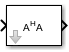# Matrix Square

Compute square of input matrix

• Library:

DSP System Toolbox / Math Functions / Matrices and Linear Algebra / Matrix Operations

•## Description

The Matrix Square block computes the square of an M-by-N input matrix, u, by premultiplying with the Hermitian transpose. The equivalent MATLAB® code is:

`y = u' * u`

The block treats length-M unoriented vector inputs as an M-by-1 matrix. When the input is an M-by-N matrix, the output of the block is an N-by-N matrix.

### Applications

The Matrix Square block can be used in a variety of applications:

• General matrix squares — The Matrix Square block computes the output matrix, y, without explicitly forming u'. It is therefore more efficient than other methods for computing the matrix square.

• Sum of squares — When the input is a column vector (N=1), the operation of the block is equivalent to a multiply-accumulate (MAC) process, or inner product. The output is the sum of the squares of the input, and is always a real scalar.

• Correlation matrix — When the input is a row vector (M=1), the output, y, is the symmetric autocorrelation matrix, or outer product.

## Ports

### Input

expand all

Input matrix, specified as an M-by-1, 1-by-N, or M-by-N matrix.

Data Types: `single` | `double` | `half` | `int8` | `int16` | `int32` | `int64` | `uint8` | `uint16` | `uint32` | `uint64` | `fixed point`

### Output

expand all

Output matrix, returned as an N-by-N or M-by-M matrix.

Data Types: `single` | `double` | `half` | `int8` | `int16` | `int32` | `int64` | `uint8` | `uint16` | `uint32` | `uint64` | `fixed point`

## Parameters

expand all

Minimum output value for range checking that Simulink® checks. Simulink software uses this value to perform:

#### Programmatic Use

 Block Parameter: `OutMin` Type: character vector Values: ```'[ ]'```| scalar Default: ```'[ ]'```

Maximum output value for range checking that Simulink checks. Simulink software uses this value to perform:

#### Programmatic Use

 Block Parameter: `OutMax` Type: character vector Values: ```'[ ]'```| scalar Default: ```'[ ]'```

Specify the output data type for this block.

You can select one of these options:

• A rule that specifies how to inherit a data type, such as `Inherit: Inherit via internal rule`. For more information on this rule, see Inherit via Internal Rule (DSP System Toolbox).

• An expression that evaluates to a valid data type, for example, `fixdt([],16,0)`

Click the buttonto display the Data Type Assistant, which helps you set the Output data type parameter. For more information, see Specify Data Types Using Data Type Assistant.

#### Programmatic Use

 Block Parameter: `OutDataTypeStr` Type: character vector Values: ```'Inherit: Same as input'``` | ```'Inherit: Inherit via internal rule'```| ```'Inherit: Inherit via back propagation'``` | `'double'` | `'single'` | `'int8'` | `'uint8'` | `int16` | `'uint16'` | `'int32'` | `'uint32'` | `'int64'` | `'uint64'` | `fixdt(1,16,0)` | `''` Default: ```'Inherit: Inherit via internal rule'```

Select this parameter to prevent the fixed-point tools from overriding the Output data type you specify on the block mask. For more information, see Use Lock Output Data Type Setting (Fixed-Point Designer).

#### Programmatic Use

 Block Parameter: `LockScale` Type: character vector Values: `'off' | 'on'` Default: `'off'`

Select the rounding mode (DSP System Toolbox) for fixed-point operations.

#### Programmatic Use

 Block Parameter: `RndMeth` Type: character vector Values: ```'Ceiling' | 'Convergent' | 'Floor' | 'Nearest' | 'Round' | 'Simplest' | 'Zero'``` Default: `'Floor'`

Select this check box to have overflows saturate to the maximum or minimum value that the data type can represent. If you clear this check box, the block wraps all overflows. See Precision and Range (DSP System Toolbox) for more information.

When you select this check box, saturation applies to every internal operation on the block, not just the output or result. The code generation process can generally detect when overflow is not possible. In this case, the code generator does not produce saturation code.

#### Programmatic Use

 Block Parameter: `SaturateOnIntegerOverflow` Type: character vector Value: `'off'` | `'on'` Default: `'off'`

## Block Characteristics

 Data Types `double` | `fixed point` | `integer` | `single` Direct Feedthrough `no` Multidimensional Signals `no` Variable-Size Signals `no` Zero-Crossing Detection `no`

## Compatibility Considerations

expand all

Behavior changed in R2021b

## Extended Capabilities

### Fixed-Point ConversionDesign and simulate fixed-point systems using Fixed-Point Designer™.

| | | | | | | | (DSP System Toolbox)

### Topics

Introduced before R2006a